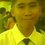# Nth Derivative of a Polynomial (part 1)

In calculus, the derivative of a polynomial is:${ ax }^{ n }={ nax }^{ n-1 }$. But how could we know the 2nd, 3rd or even the 100th derivative of a given polynomial expression. I formulated new formulas that would ensure that the time in finding the number of derivative would be less in calculating the higher derivatives. These are the following conditions:

Let's assume that n is the no. of differentiation or derivatives that a polynomial must derive and b be the exponents of each term in a given polynomial.

1. If $n , then the nth derivative of the polynomial would be

$\frac { { d }^{ n } }{ { dx }^{ n } } { ax }^{ b }=a(b-n+1)(b-n+2)....(b-n+n){ x }^{ b-n }$.

Example: Find the third derivative of ${ 4x }^{ 5 }$?

Solution: Since the condition is $n then let's use the formula stated in first condition. Let n be 3 and b be 5.Therefore, the solution would be:

$\frac { { d }^{ 3 } }{ { dx }^{ 3 } } { 4x }^{ 5 }=4(5-3+1)(5-3+2)(5-3+3){ x }^{ 5-3 }$

$\frac { { d }^{ 3 } }{ { dx }^{ 3 } } { 4x }^{ 5 }=4(3)(4)(5){ x }^{ 5-3 }$

$\frac { { d }^{ 3 } }{ { dx }^{ 3 } } { 4x }^{ 5 }={ 240x }^{ 2 }$Note by Merzel Mark Guilaran
6 years, 5 months ago

This discussion board is a place to discuss our Daily Challenges and the math and science related to those challenges. Explanations are more than just a solution — they should explain the steps and thinking strategies that you used to obtain the solution. Comments should further the discussion of math and science.

When posting on Brilliant:

• Use the emojis to react to an explanation, whether you're congratulating a job well done , or just really confused .
• Ask specific questions about the challenge or the steps in somebody's explanation. Well-posed questions can add a lot to the discussion, but posting "I don't understand!" doesn't help anyone.
• Try to contribute something new to the discussion, whether it is an extension, generalization or other idea related to the challenge.
• Stay on topic — we're all here to learn more about math and science, not to hear about your favorite get-rich-quick scheme or current world events.

MarkdownAppears as
*italics* or _italics_ italics
**bold** or __bold__ bold
- bulleted- list
• bulleted
• list
1. numbered2. list
1. numbered
2. list
Note: you must add a full line of space before and after lists for them to show up correctly
paragraph 1paragraph 2

paragraph 1

paragraph 2

[example link](https://brilliant.org)example link
> This is a quote
This is a quote
    # I indented these lines
# 4 spaces, and now they show
# up as a code block.

print "hello world"
# I indented these lines
# 4 spaces, and now they show
# up as a code block.

print "hello world"
MathAppears as
Remember to wrap math in $$ ... $$ or $ ... $ to ensure proper formatting.
2 \times 3 $2 \times 3$
2^{34} $2^{34}$
a_{i-1} $a_{i-1}$
\frac{2}{3} $\frac{2}{3}$
\sqrt{2} $\sqrt{2}$
\sum_{i=1}^3 $\sum_{i=1}^3$
\sin \theta $\sin \theta$
\boxed{123} $\boxed{123}$

## Comments

Sort by:

Top Newest

Yes in general $\dfrac{d^n}{dx^n} (ax^r) = an! {\dbinom{r}{n}} x^{r-n}$. Good insight by the way sir.

- 3 years, 2 months ago

Log in to reply

×

Problem Loading...

Note Loading...

Set Loading...